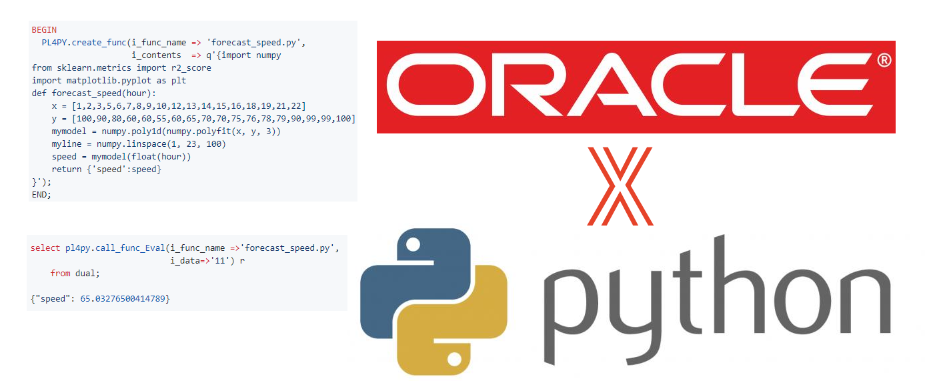# 【ORACLE】在oracle10g以上版本启用数据库plsql对python函数的支持（pl4py）

## 2021-11-01 更新，增加linux环境支持

【ORACLE】pl4py更新，支持linux环境（在Oracle中调用python函数并获得返回值）

# pl4py

（不是21c版本中的OML4PY 【ORACLE】在ORACLE数据库中启用机器学习功能（OML）以支持PYTHON脚本的运行

## 安装步骤

#### 第一步

``````pip3 install flask
``````

#### 第二步

``````mkdir F:\oracle\PY_FILE
``````

#### 第三步

``````create or replace directory PY_FILE as 'F:\oracle\PY_FILE'
``````

#### 第四步

``````grant select on dba_directories to {username} with grant option;
``````

#### 第五步

• PL4PY_FUNC_LIST.sql
• PL4PY_FUNC_LIST_TRI.trg
• PL4PY.pck
• pl4py_func_list_v.sql

## 使用举例

#### 一、启动服务

``````begin
PL4PY.start_service;
end;
``````

#### 二、创建（或更新）函数

``````BEGIN
PL4PY.create_func(i_func_name => 'sample_f.py',
i_contents  => q'{def sample_f(num):
return float(num)*float(num)
}');
END;
``````

#### 三、使用函数

``````select  pl4py.call_func_Eval('sample_f.py', '8') r from dual;

64
``````

#### 四、删除函数

``````BEGIN
PL4PY.drop_func(i_func_name => 'sample_f.py');
END;
``````

#### 五、停止服务

``````begin
PL4PY.stop_service;
end;
``````

## 更复杂的函数例子

1. 基于原始数据计算一个时间和速度相关性的模型，然后输入时间，返回速度
``````BEGIN
PL4PY.create_func(i_func_name => 'forecast_speed.py',
i_contents  => q'{import numpy
from sklearn.metrics import r2_score
import matplotlib.pyplot as plt
def forecast_speed(hour):
x = [1,2,3,5,6,7,8,9,10,12,13,14,15,16,18,19,21,22]
y = [100,90,80,60,60,55,60,65,70,70,75,76,78,79,90,99,99,100]
mymodel = numpy.poly1d(numpy.polyfit(x, y, 3))
myline = numpy.linspace(1, 23, 100)
speed = mymodel(float(hour))
return {'speed':speed}
}');
END;

select pl4py.call_func_Eval(i_func_name =>'forecast_speed.py', i_data=>'11') r from dual

{"speed": 65.03276500414789}
``````
1. 传入一个sql，获得sql中的所有表或视图名称
https://github.com/Dark-Athena/list_table_sql-py
中的所有文件下载到安装步骤第二步中的文件夹，比如 “F:\oracle\PY_FILE”
然后在数据库中以实际文件的方式创建函数
``````BEGIN
PL4PY.create_func(i_func_name => 'list_table_sql.py',
i_dir  => 'PY_FILE',
i_file_name  =>'list_table_sql.py');
END;

declare
r varchar2(4000);
i_data varchar2(4000);
begin
i_data:='{"sql":"select abc from def,ghi j,k.lmn o","mode":"T"}';
r := pl4py.call_func_Eval(i_func_name =>'list_table_sql.py', i_data=>i_data);
dbms_output.put_line(r);
end;

{"tablename": ["def", "ghi", "k.lmn"]}
``````

## 注意事项:

1. 服务被设计成长期运行的schedule_job，函数动态切换，因此除非要完全停止或者检查问题，一般不需要执行 PL4PY.stop_service
2. i_func_name参数必须带后缀 ".py"
3. 视图 "pl4py_func_list_v"提供自定义python函数脚本内容的查询++++++++